# Why Rationals Won’t Cure Your Floating Point Blues

Why Rationals Won’t Cure Your Floating Point Blues

By Richard Harris

Numerical computing is still proving hard to do accurately. Richard Harris considers another number representation.

In the first article in this series we described floating point arithmetic and noted that its oft criticised rounding errors are relatively inconsequential in comparison to the dramatic loss of precision than results from subtracting two almost equal numbers. We demonstrated that the order in which we perform operations, whilst irrelevant for real numbers, can affect the result of a floating point expression and that consequently we must be careful how we construct expressions if we wish their results to be as accurate as possible.

In the second article we discussed the commonly proposed alternative of fixed point numbers and found that, although it is supremely easy to reason about addition and subtraction when using them, they can suffer even more greatly than floating point numbers from truncation error, cancellation error and order of execution.

## Rationals

So, can we do any better?

Perhaps if we were to implement a rational number type, in which we explicitly maintain both the numerator and the denominator, rather than declare by fiat that we are working to some fixed number of decimal places or significant figures.

The rules of rational arithmetic are pretty straightforward. Given two rationals a 0 / b 0 and a 1/ b 1 we have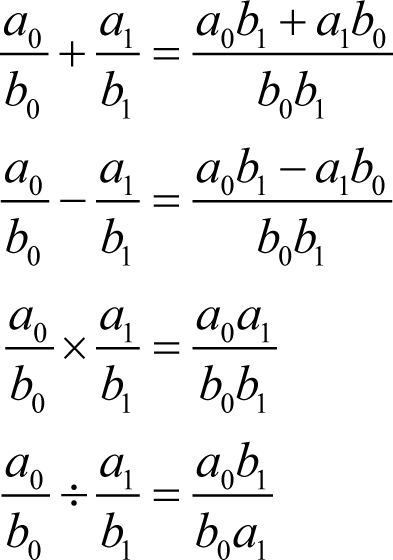One enormous advantage of rational numbers is that, provided we do not overflow the integers representing the numerator (the top of the fraction) and the denominator (the bottom) the order of execution of these arithmetic operations is irrelevant; the answer will always be the same. Given that we have gone to great lengths to create an integer type that cannot overflow, this behaviour will prove rather useful.

The only thing we need to watch out for is the fact that there are many ways of writing down the same number; 1/2, 2/4 and 3/6 all represent the same number, for example. We shall ensure that our representation is unique by insisting that the numerator and the denominator are the smallest numbers that yield the same rational, or equivalently have no common factors, and that the denominator is positive.

The latter condition is relatively straightforward to maintain. The former requires an algorithm to determine the highest common factor, or HCF, of a pair of numbers, the greatest positive integer that wholly divides both. We can subsequently divide out that factor and return any rational to its simplest form. Fortunately one such algorithm has been handed down to us from antiquity, courtesy of the great Euclid and it proceeds as follows.

## Euclid’s algorithm

If the two numbers are equal, their value is the HCF.

If the smaller exactly divides the larger, the smaller is the HCF.

Otherwise, divide the larger by the smaller, and make note of the remainder. The HCF of the original numbers is equal to the HCF of the smaller number and the remainder.

In mathematical notation this can be expressed as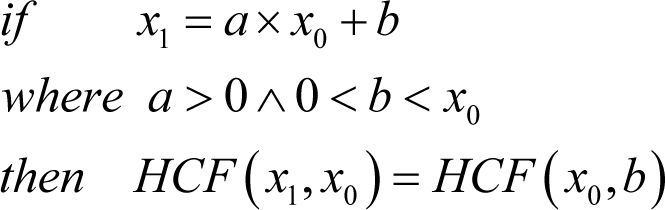Recursively applying these rules is guaranteed to terminate and we can thus determine the HCF.

For example, applying the Euclidean algorithm to 2163 and 1785 yields the following steps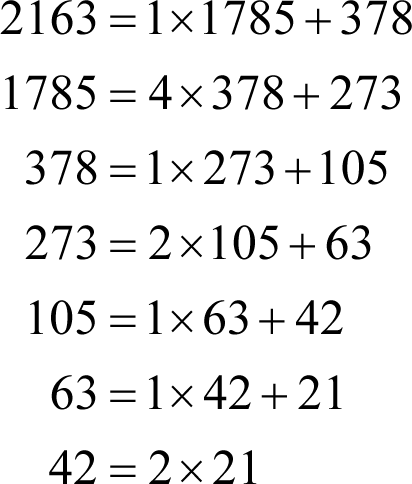and hence the HCF of 2163 and 1785 is 21, a fact that is clear if we look at their prime factorisations.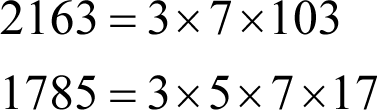As it happens, this is simply a special case of the more general result that for any integers x 0 , x 1 , a and b where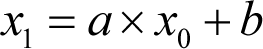then x 0 and b must have the same highest common factor as x 0 and x 1 , as shown in derivation 1.

Proof of common shared factors

First, let us assume that x 0 and x 1 share a common factor of c . We can therefore rewrite the equation as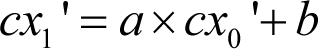for some x 0 ' and x 1 '.

Now since the left hand side is wholly divisible by c then so must the right hand side and furthermore since the first term on the right hand side is wholly divisible by c then so must be the second term, allowing us to express the equation as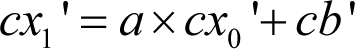Second, let us assume that x 0 and b share a different common factor of d . We can now rewrite the equation as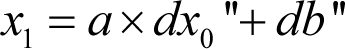for some x 0 " and b ".

But now the right hand side is wholly divisible by d and so therefore must be the left hand side.

Hence any factor shared by x 0 and x 1 must be shared by x 0 and b , and any factor shared by x 0 and b must be shared by x 0 and x 1 and that they must consequently have the exactly the same highest common factor.

Derivation 1

As a consequence, it should not be surprising that the algorithm converges faster if we round the result of the division to the nearest integer rather than round down, consequently admitting negative remainders, and use the absolute value of the remainder in the following step.

Applying this optimisation to the same pair of numbers yields the same result in fewer steps.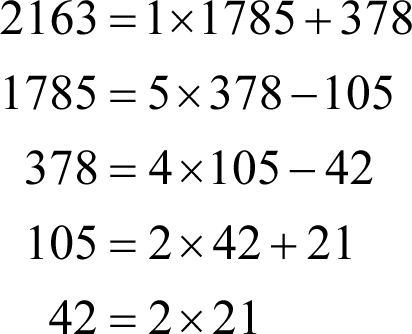## A rational class

Now that we have described the various arithmetic operations, and the scheme that we shall use to ensure that each rational has a unique representation, we are ready to actually implement it. Listing 1 illustrates the class definition of our rational number type.

 ```template class rational { public: typedef T term_type; rational(); rational(const term_type &x); rational(const term_type &numerator, const term_type &denominator); const term_type & numerator() const; const term_type & denominator() const; int compare(const rational &x) const; rational & negate(); rational & operator+=(const rational &x); rational & operator-=(const rational &x); rational & operator*=(const rational &x); rational & operator/=(const rational &x); private: rational & normalise(); term_type numerator_; term_type denominator_; }; ``` Listing 1

The first thing we shall need is a helper function to compute the HCF of a pair of positive integers as given in listing 2.

 ```template T hcf(T x0, T x1) { if(x0<=0 || x1<=0) throw std::invalid_argument(""); if(x0

Note that we capture both termination conditions by checking whether the absolute remainder, now stored in x 1 , is equal to 0. This will be true both if the smaller number is equal to or wholly divides the larger.

We implement the more efficient version of the algorithm by checking whether the remainder is greater than half the divisor. If it is, then the absolute value of the remainder of the rounded closest, rather than rounded down, division is simply the divisor minus the remainder.

We can see that this is true by considering the implications on the remainder of increasing the result by 1. In mathematical notation, the initial step is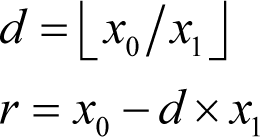where the odd looking brackets mean the largest integer less than or equal to their contents.

The new remainder is equal to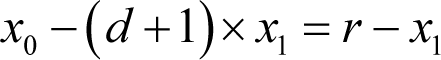which is guaranteed to be negative, meaning that the absolute value of the new remainder must be x 1 r .

We could improve performance a little for ``` bignum ``` s by overloading this function to exploit the fact that their division helper function also calculates the remainder. However, since our division algorithm is O ( n 2 ) in the number of bits and our multiplication algorithm is only O ( n 2 ) in the number of digits, it would probably not make that much difference in most cases.

Next we shall implement the ``` normalise ``` member function which we shall use to ensure that our ``` rational ``` s are always represented in a standard form, as shown in listing 3. In this form, common factors are removed, the denominator is always positive and shall furthermore be equal to 1 when the numerator is 0.

 ```template rational & rational::normalise() { if(denominator_==0) throw std::invalid_argument(""); if(denominator_<0) { numerator_ = -numerator_; denominator_ = -denominator_; } if(numerator_==0) { denominator_ = 1; } else { const T c = hcf(abs(numerator_), denominator_); numerator_ /= c; denominator_ /= c; } return *this; } ``` Listing 3

We shall first call this function in one of the constructors, as given in listing 4. Specifically, we shall not entrust the correct representation to the user when construction from numerator and denominator.

 ```template rational::rational() : numerator_(0), denominator_(1) { } template rational::rational(const term_type &x) : numerator_(x), denominator_(1) { } template rational::rational(const term_type &numerator, const term_type &denominator) : numerator_(numerator), denominator_(denominator) { normalise(); } ``` Listing 4

The remaining member functions are equally straightforward which should come as no surprise given the simplicity of rational arithmetic.

The data access member functions, ``` numerator ``` and ``` denominator ``` , together with the ``` compare ``` and ``` negate ``` member functions are shown in listing 5.

 ```template const rational::term_type & rational::numerator() const { return numerator_; } template const rational::term_type & rational::denominator() const { return denominator_; } template int rational::compare(const rational &x) const { const term_type lhs = numerator_ * x.denominator_; const term_type rhs = denominator_ * x.numerator_; if(lhsrhs) return 1; return 0; } template rational & rational::negate() { numerator_ = -numerator_; return *this; } ``` Listing 5

Note that we must multiply the numerators and denominators during comparison which, for fixed width integer types, introduces the possibility of overflow and, for ``` bignum ``` s, unfortunately makes it a relatively costly operation.

The arithmetic operators, given in listing 6, are similarly sensitive to overflow when using fixed width integers and similarly expensive when using ``` bignum ``` s. Most irritating is that fact that addition and subtraction are now more sensitive to these factors than multiplication and division.

 ```template rational & rational::operator+=(const rational &x) { numerator_ = numerator_ * x.denominator_ + denominator_ * x.numerator_; denominator_ *= x.denominator_; return normalise(); } template rational & rational::operator-=(const rational &x) { numerator_ = numerator_ * x.denominator_ - denominator_ * x.numerator_; denominator_ *= x.denominator_; return normalise(); } template rational & rational::operator*=(const rational &x) { numerator_ *= x.numerator_; denominator_ *= x.denominator_; return normalise(); } template rational & rational::operator/=(const rational &x) { numerator_ *= x.denominator_; denominator_ *= x.numerator_; return normalise(); } ``` Listing 6

## The problem with rationals

Recall that I mentioned that the square root of 2 is irrational and hence cannot be equal to any integer divided by another. A demonstration of this fact is given in derivation 2.

Proving that the square root of 2 is not rational

Let us assume that there are integers a and b such that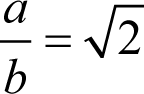and that we have cancelled all common factors so that their HCF is 1. Trivially, we have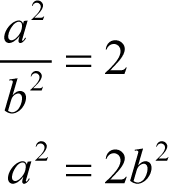Now any odd number multiplied by itself results in another odd number, so a must be even and hence equal to 2 a ' for some a '. Hence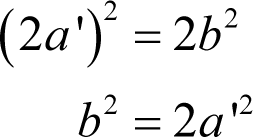But this similarly means that b must be even and that consequently a and b have a common factor of 2; a contradiction.

The square root of 2 cannot, therefore, be rational.

Keep it to yourself though; you might get drowned.

Derivation 2

We cannot therefore exactly represent any such number with our ``` rational ``` type. However, it is also true that for every irrational number there are an infinite number of rationals to be found within any given positive distance, no matter how small.

Perhaps we could represent an irrational with one of its rational neighbours?

Well, yes we could, but we’d have to decide exactly how distant that rational should be and, whatever distance we choose, we could match its accuracy with a floating point representation of sufficient precision.

So, whilst rational number types are supremely accurate for addition, subtraction, multiplication and division and are consequently not sensitive to the order of execution of these operations, they require no less care and attention than floating point number types the instant we start mucking about with non-linear equations.

I am reluctant to categorise this capable numeric type as a lame duck, but am compelled to observe that, so far as general purpose arithmetic is concerned, it does seem to have a pronounced limp.

Quack, quack, quack.